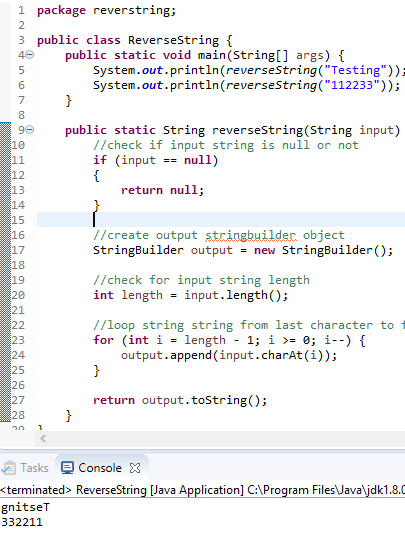In our previous article, we have provided list of Java pattern programs for beginners but in this article, I am going to provide some simple java programs examples for core java practice, which can be useful for beginners to learn Java basics.

### Swapping two numbers without using third variable

``````public static void main(String[] args) {
int first = 11, second = 15;

System.out.println("--Before swap--");
System.out.println("First number = " + first);
System.out.println("Second number = " + second);

first = first - second;
second = first + second;
first = second - first;

System.out.println("--After swap--");
System.out.println("First number = " + first);
System.out.println("Second number = " + second);

}``````

Output:

``````--Before swap--
First number = 11
Second number = 15
--After swap--
First number = 15
Second number = 11``````

In the above code, we are initially subtracting number numbers, to save remainder in first variable (11-15 = -4) and then adding both numbers to save value in second variable (-4 + 15=11).

Once we have implemented above, steps, second variable already is swapped, we just now we just need to subtract (11 - (-4) =15), which gives our required result.### Program to reverse a string

``````package reverstring;

public class ReverseString {
public static void main(String[] args) {
System.out.println(reverseString("Testing"));
System.out.println(reverseString("112233"));
}

public static String reverseString(String input) {
//check if input string is null or not
if (input == null)
{
return null;
}

//create output stringbuilder object
StringBuilder output = new StringBuilder();

//check for input string length
int length = input.length();

//loop string string from last character to first and save it in output string
for (int i = length - 1; i >= 0; i--) {
output.append(input.charAt(i));
}

return output.toString();
}
}
``````

Output

``````gnitseT
332211``````

In the above code, we have created a seperate function to reverse the string.

In that function we are initially cheking if string is `null` or not, once we are confirmed string is not `null`, we are looping through string characters one by one starting from last character first and first character in the end, and saving it in output `StringBuilder` object.### Program to check if number is prime or not in Java

Prime numbers are those numbers which are divisble by itself only, so if number is divided by any other number then it is not a prime.

``````package primenumber;
import java.util.*;

public static void main(String args[]){
int num,loop;
boolean IsPrimeFlag=false;

Scanner GetUserInput=new Scanner(System.in);

System.out.println("Enter any integer number: ");
num= GetUserInput.nextInt();

//check if number is prime
for(loop=2; loop<=(num/2); loop++)
{
if(num%loop==0)
{
IsPrimeFlag=true;
break;
}
}

if(IsPrimeFlag==false)
{
System.out.println(num + " is a prime number.");
}
else
{
System.out.println(num + " is not a prime number.");
}

}
}
``````

Output:

``````Enter any integer number:
13
13 is a prime number.

Enter any integer number:
55
55 is not a prime number.
``````

### Factorial Program in Java

A factorial is a function that multiplies number by every number until 1.

For example 4!= 4*3*2*1=24. The function is used, among other things, to find the number of ways "n" objects can be arranged.

``````public class factorial
{
public static void main(String arg[])
{
int n=5,fact=1;

//loop though all number until the number is hit
for(int i=1;i<=n;i++)
{
//multiple by current number
fact=fact*i;
}

System.out.println("factoral="+fact);
}
}``````

Output:

``factorial =120``

### Fibonacci Series Program in Java

``````public class Fibonacci{
{
public static void main(String[] args){
Main  fib = new Main();
fib.fibonacci(10);
}
public void fibonacci(int num){
int a=0, b=1;
int c;
System.out.print(a + " "+b );

//loop through numbers, below 'num'
for(int i=2;i<num;i++){
c = a+b;
a = b;
b = c;
System.out.print(" "+c);
}
}

//recursive method to add last 2 numbers
public int recFibonacci(int a, int b){
int c =a+b;
return c;
}

}``````

Output:

``0 1 1 2 3 5 8 13 21 34``

In the above code, we are using Recursion to call `recFibonacci` method again and again, until our required position number is not hit by loop.

And for each call, the last numbers are added and printed by Java code.

### Sort an array in Java

``````package sort;

public class SortArray {
public static void main(String args[]) {
int[] array = { 2, 3, 4, 5, 3, 4, 2, 34, 56, 98, 32, 54 };
boolean swapped = true;
int j = 0;
int tmp;
//start swapping until all values are not swapped
while (swapped) {
swapped = false;
j++;
//loop all values
for (int i = 0; i < array.length - j; i++) {
//if adjacent value is greater than current then swap positions
if (array[i] > array[i + 1]) {
tmp = array[i];
array[i] = array[i + 1];
array[i + 1] = tmp;
swapped = true;
}
}
}

//print sorted array
for(int i=0;i<array.length;i++)
{
System.out.print(array[i] + " ");
}
}
}
``````

Output:

``2 2 3 3 4 4 5 32 34 54 56 98 ``

In the above code, I have tried to explained the code using comments, we are using Bubble sort in the above code to sort the array.

### Matrix Multiplication Program in Java

``````package matrixmulti;

public class MatrixMultiplication {
static int b[][]={{21,21},{22,22}};

static int a[][] ={{1,1},{2,2}};
public static void main(String args[]){

maxtriMultiply();
}

public static void maxtriMultiply(){
int c[][] = new int;

for(int i=0;i<b.length;i++){
for(int j=0;j<b.length;j++){
c[i][j] =0;
}
}

for(int i=0;i<a.length;i++){
for(int j=0;j<b.length;j++){
for(int k=0;k<b.length;k++){
c[i][j]= c[i][j] +(a[i][k] * b[k][j]);
}
}
}

for(int i=0;i<c.length;i++){
for(int j=0;j<c.length;j++){
System.out.print(c[i][j]);
}
System.out.println("\n");
}
}

}
``````

Output:

``````4343

8686
``````

You may also like to read:

Java Pattern Program Examples